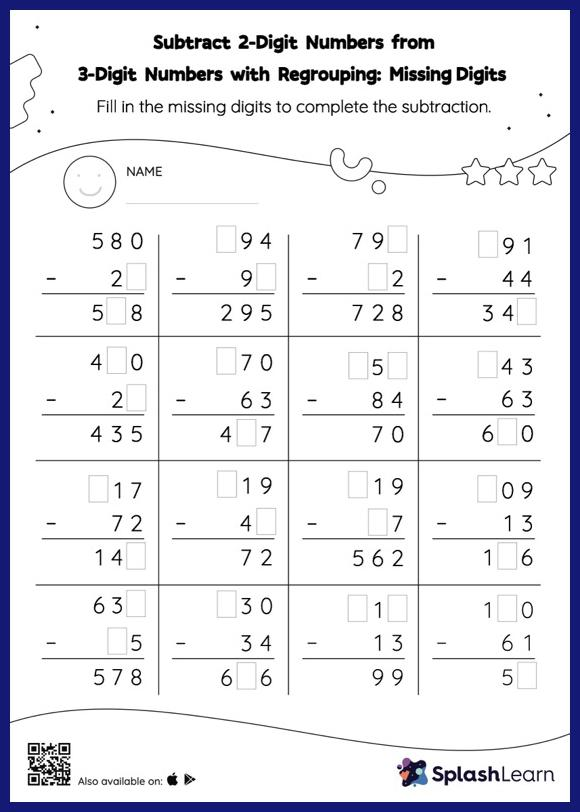# Subtract 2-Digit Numbers from 3-Digit Numbers with Regrouping: Missing Digits Worksheet

Home > Subtract 2-Digit Numbers from 3-Digit Numbers with Regrouping: Missing DigitsGive your little one the opportunity to dive deeper into subtraction with this subtract 2-digit numbers from 3-digit numbers with regrouping worksheet. To find the missing number on this worksheet, students can utilize the count back strategy or the connection between addition and subtraction. In subtract 2-digit numbers from 3-digit numbers with regrouping worksheet, they must additionally regroup numbers to reach the solution. This worksheet is about practicing the column method in which numbers are written one on top of another. In this method, students use their place value understanding to solve the problems.